E6 Next: E7 Up: Exceptional Lie Algebras Previous: The Magic Square

## 4.4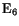We begin with the 78-dimensional exceptional Lie group. As we mentioned in Section 3.4, there is a nice description of a certain noncompact real form ofas the group of collineations of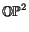, or equivalently, the group of determinant-preserving linear transformations of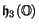. But before going into these, we consider the magic square constructions of the Lie algebra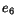. Vinberg's construction gives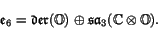Tits' construction, which is asymmetrical, gives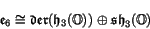and also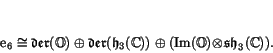The Barton-Sudbery construction gives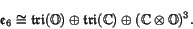We can use any of these to determine the dimension of. For example, we have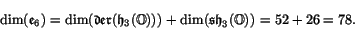Starting from the Barton-Sudbery construction and using the concrete descriptions of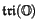and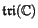from equation (3), we obtain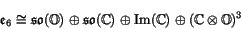Using equation (4.2), we may rewrite this as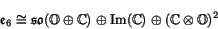and it turns out that the summand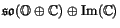is actually a Lie subalgebra of. This result can also be found in Adams' book , phrased as follows: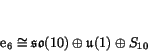In fact, he describes the bracket inin terms of natural operations involving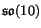and its spinor representation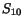. The funny-looking factor of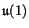comes from the fact that this spinor representation is complex. The bracket of an element ofand an element ofis another element of, defined using the obvious action ofon this complex vector space.

If we defineto be the simply connected group with Lie algebra, it follows from results of Adams that the subgroup generated by the Lie subalgebra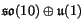is isomorphic to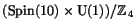. This lets us define the bioctonionic projective plane by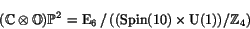and conclude that the tangent space at any point of this manifold is isomorphic to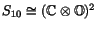.

Sinceis compact, we can put an-invariant Riemannian metric on the bioctonionic projective plane by averaging any metric with respect to the action of this group. It turns out  that the isometry group of this metric is exactly, so we have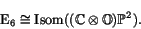It follows that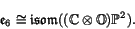Summarizing, we have 6 octonionic descriptions of:

Theorem 6.   The compact real form ofis given by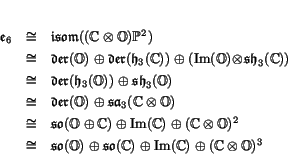where in each case the Lie bracket ofis built from natural bilinear operations on the summands.

The smallest nontrivial representations ofare 27-dimensional: in fact it has two inequivalent representations of this dimension, which are dual to one another. Now, the exceptional Jordan algebra is also 27-dimensional, and in 1950 this clue led Chevalley and Schafer  to give a nice description ofas symmetries of this algebra. These symmetries do not preserve the product, but only the determinant.

More precisely, the group of determinant-preserving linear transformations ofturns out to be a noncompact real form of. This real form is sometimes called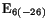, because its Killing form has signature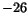. To see this, note that any automorphism ofpreserves the determinant, so we get an inclusion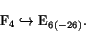This means that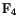is a compact subgroup of. In fact it is a maximal compact subgroup, since if there were a larger one, we could average a Riemannian metric group onwith respect to this group and get a metric with an isometry group larger than, but no such metric exists. It follows that the Killing form on the Lie algebra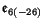is negative definite on its 52-dimensional maximal compact Lie algebra,and positive definite on the complementary 26-dimensional subspace, giving a signature of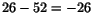.

We saw in Section 3.4 that the projective plane structure ofcan be constructed starting only with the determinant function on the vector space. It follows thatacts as collineations on, that is, line-preserving transformations. In fact, the group of collineations ofis precisely: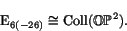Moreover, just as the group of isometries offixing a specific point is a copy of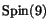, the group of collineations fixing a specific point is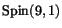. This fact follows with some work starting from equation (3.4), and it gives us a commutative square of inclusions: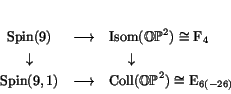where the groups on the top are maximal compact subgroups of those on the bottom. Thus in a very real sense,is to 9-dimensional Euclidean geometry asis to 10-dimensional Lorentzian geometry.

Next: E7 Up: Exceptional Lie Algebras Previous: The Magic Square